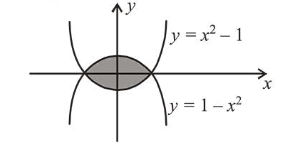# The area (in sq. units) of the region enclosed by the curves

Question:

The area (in sq. units) of the region enclosed by the curves $y=x^{2}-1$ and $y=1-x^{2}$ is equal to:

1. (1) $\frac{4}{3}$

2. (2) $\frac{8}{3}$

3. (3) $\frac{7}{2}$

4. (4) $\frac{16}{3}$

Correct Option: , 2

Solution:

Required areaArea $=2 \int_{0}^{1}\left(\left(1-x^{2}\right)-\left(x^{2}-1\right)\right) d x=4 \int_{0}^{1}\left(1-x^{2}\right) d x$

$=\left.4\left(x-\frac{x^{3}}{3}\right)\right|_{0} ^{1}=4\left(1-\frac{1}{3}\right)=4 \cdot \frac{2}{3}=\frac{8}{3}$ sq. units